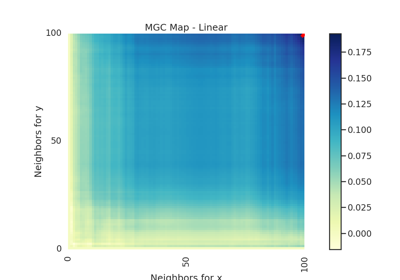# spiral¶

hyppo.tools.spiral(n, p, noise=False, low=0, high=5)

Spiral simulation.

Spiral $$(X, Y) \in \mathbb{R}^p \times \mathbb{R}$$: $$U \sim \mathcal{U}(0, 5)$$, $$\epsilon \sim \mathcal{N}(0, 1)$$

$\begin{split}X_{|d|} &= U \sin(\pi U) \cos^d(\pi U)\ \mathrm{for}\ d = 1,...,p-1 \\ X_{|p|} &= U \cos^p(\pi U) \\ Y &= U \sin(\pi U) + 0.4 p \epsilon\end{split}$
Parameters
Returns

x,y (ndarray) -- Simulated data matrices. x and y have shapes (n, p) and (n, 1) where n is the number of samples and p is the number of dimensions.

## Examples using hyppo.tools.spiral`¶